### INTRODUCTION¶

There are times when we unwittingly commit the same mistake incessantly without actually discovering the reason being it because we never fathom that something of that sort can actually happen. One of first hands-on application that fledgling Computer Vision enthusiasts start with is color detection to get the pixel locations with the desired color (and yes, OpenCV function cv2.inRange performs this task). So, in this notebook, I will discuss about one of the many mistake most newbies commit. I did commit this peccadillo for a long time before I could figure it out. Making mistakes is not a mistake, but not correcting them is definitely. The philosopher in me, sometimes overpowers the engineer in me. Leaving me aside, in this IPython Notebook we will try to detect the Manchester United jersey in the image below using color detection. I have chosen the red jersey intentionally and you will figure it out why I did so as we go through this tutorial.

### IMPLEMENTATION¶

The first step is to import the necessary modules -

1. pylab - We will use pylab to display the images
2. cv2 - We will use cv2 function to manipulate our images.
In :
%pylab inline
import cv2

Populating the interactive namespace from numpy and matplotlib


We will define a function named myShow to display the image.

In :
def myShow(im_title, im):
''' This is function to display the image'''
plt.figure()
plt.title(im_title)
plt.axis("off")
if len(im.shape) == 2:
plt.imshow(im, cmap = "gray")
else:
im_display = cv2.cvtColor(im, cv2.COLOR_RGB2BGR)
plt.imshow(im_display)
plt.show()


We will read a image and display it using the myShow function.

In :
im = cv2.imread("manu.jpg")
myShow("Input Image", im)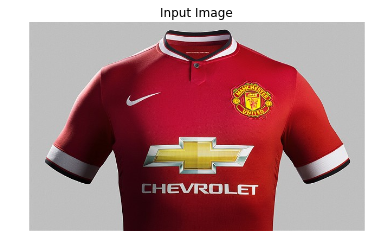As you can notice, the jersey is predominantly red, so we will set the cv2.inrange function with the range of RGB values of the Red color. The code snippet below performs this task -

In :
im_red_ball_mask = cv2.inRange(im, (0, 0, 30), (80, 80, 255))
im_red_ball_mask = im_red_ball_mask.astype('bool')
myShow("Resulting Image", im * np.dstack((im_red_ball_mask, im_red_ball_mask, im_red_ball_mask)))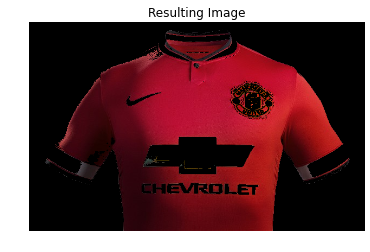If you look meticulously, you will notice that there are some white pixels that have been incorrectly classified as Red pixels. In order to remove these pixels, we will perform color detection in the HSV color space. Let's convert the image into HSV format using the cv2.cvtColor function.

In :
im_hsv = cv2.cvtColor(im, cv2.COLOR_BGR2HSV)


The HSV values for true Red are $(0, 255, 255)$. But, in real world images there is always variations in the image colors values. So, we will use the cv2.inRange to generate the mask that has a value of $255$ for pixels where the HSV values fall in the range $(0-10, 100-255, 0-255)$ and a value of $0$ for pixels whose values don't lie in this interval. The mask values are either $255$ or $0$. $255$ represents Red color pixels and $0$ represents non Red color pixels. We will again convert this mask into boolean type and will then display the image using myShow function.

In :
im_red_ball_mask_1 = cv2.inRange(im_hsv, (0, 100, 0), (10, 255, 255))
im_red_ball_mask_1 = im_red_ball_mask_1.astype('bool')
myShow("Resulting image with Hue values within 0-10", im * np.dstack((im_red_ball_mask_1, im_red_ball_mask_1, im_red_ball_mask_1)))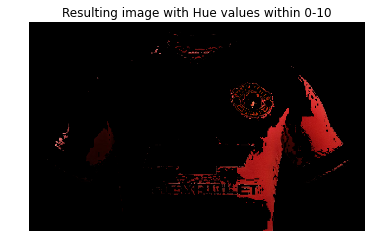But what just happened!! Instead of improving, on the contrary our results deteriorated. The resulted image does not contains a major chunk of Red Pixels that were present in the previous image. The next thought that comes to mind is to tweak the HSV Values, but believe me that would not help. I am not being a pessimist here but there is an underlying idea that needs to be understood before trying anything new. The HSV color space unlike the RGB color space is a cylindrical color space (check the image here). The Hue values are across a circle. So, after completing one rotation across the circle, we get the same color i.e. the Hue values at 0 and 360 represent the same color which happens to be Red. Just to avoid confusion w.r.t. to the diagram below -- OpenCV uses HSV ranges between $(0-180, 0-255, 0-0255)$, and what you will find in most books and the diagram below is that the range of $(0-360, 0-1, 0-1)$ is used to represent the entire gamut of HSV color space. So in OpenCV, the H values 179, 178, 177 and so on are as close to the true Red as H value 1, 2, 3 and so on.

So, I think you have grasped the concept quite well and by now, you would have guessed what we will do next. Yes, we will again use the inRange function but this time the range of Hue will be between $170-180$ instead of $0-10$ used earlier. Here is the our new code.

In :
im_red_ball_mask_2 = cv2.inRange(im_hsv, (170, 100, 0), (180, 255, 255))
im_red_ball_mask_2 = im_red_ball_mask_2.astype('bool')
myShow("Resulting image with Hue values within 170-180", im * np.dstack((im_red_ball_mask_2, im_red_ball_mask_2, im_red_ball_mask_2)))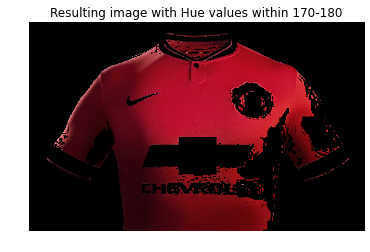That's it, we have successfully recovered our missing red color pixels by using the new H values and have also negated the white noise pixels that we had while working in the RGB color space. Now, just for the sake of aesthetics, we will combine both the masks and then we will display the final image.

In :
im_red_ball_mask_full = im_red_ball_mask_1 + im_red_ball_mask_2
myShow("Resulting image by adding both the masks", im * np.dstack((im_red_ball_mask_full, im_red_ball_mask_full, im_red_ball_mask_full)))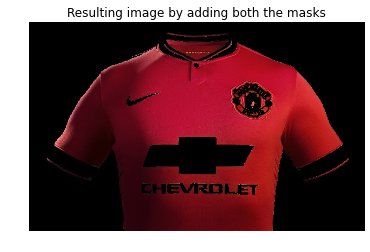### RECAP¶

In this IPython Notebook, I showed you how an oversight while selecting your Hue values for Red color can fail your code. We also discussed how to correctly select the Hue range for Red Color. You will need to keep this in mind while working in HLS space also.